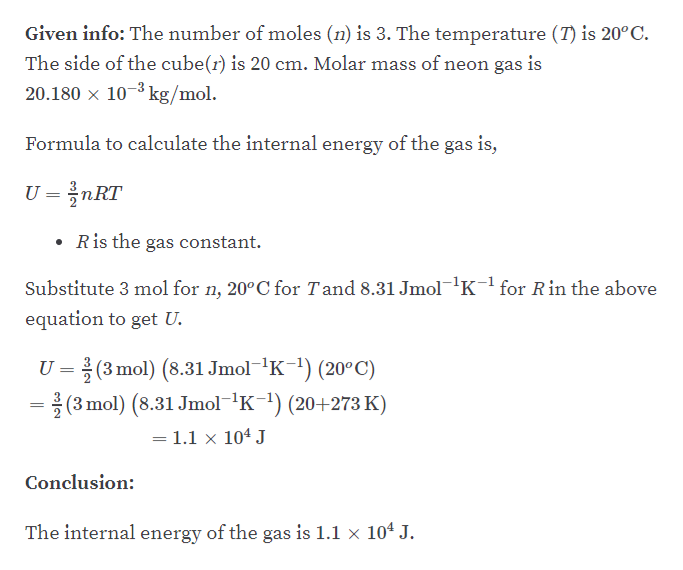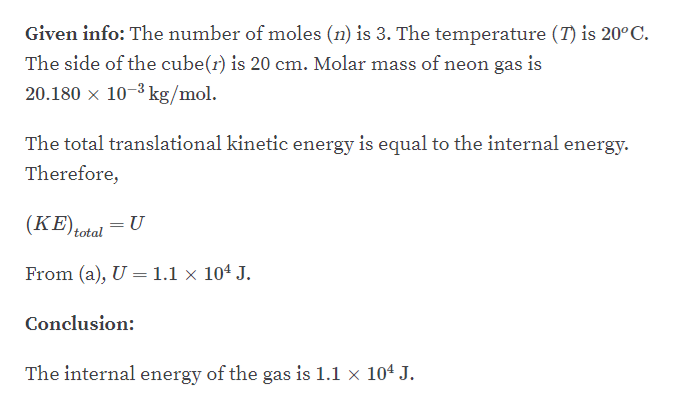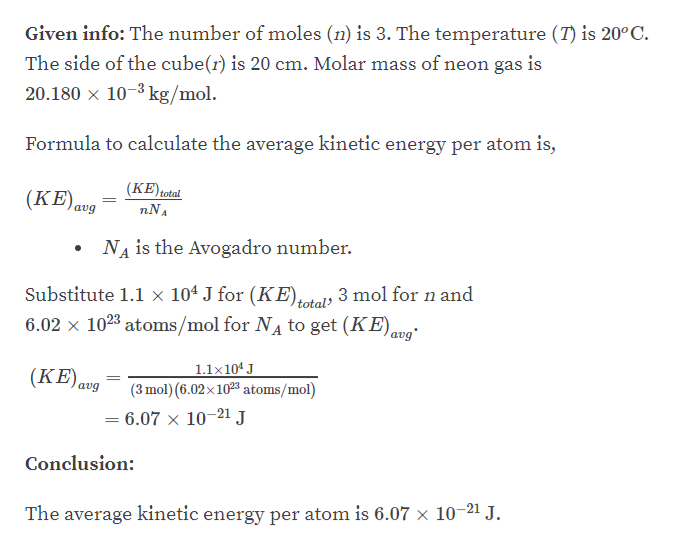# A sealed cubical container 20.0 cm on a side contains a gas with three times Avogadro’s number of neon atoms at a temperature of 20.0°C. (a) Find the internal energy of the gas. (b) Find the total translational kinetic energy of the gas.(c) Calculate the average kinetic energy per atom. (d) Use Equation 10.13 to calculate the gas pressure. (e) Calculate the gas pressure using the ideal gas law (Eq. 10.8).

Question
1 views

A sealed cubical container 20.0 cm on a side contains a gas with three times Avogadro’s number of neon atoms at a temperature of 20.0°C. (a) Find the internal energy of the gas. (b) Find the total translational kinetic energy of the gas.(c) Calculate the average kinetic energy per atom. (d) Use Equation 10.13 to calculate the gas pressure. (e) Calculate the gas pressure using the ideal gas law (Eq. 10.8).

check_circle

Step 1

Part A:help_outlineImage TranscriptioncloseGiven info: The number of moles (n) is 3. The temperature (T) is 20°C. The side of the cube(r) is 20 cm. Molar mass of neon gas is 20.180 x 10-3 kg/mol. Formula to calculate the internal energy of the gas is, U = žnRT Ris the gas constant. Substitute 3 mol for n, 20°C for Tand 8.31 Jmol1K¯l for Rin the above equation to get U. U = (3 mol) (8.31 Jmol-'K-1) (20°C) (3 mol) (8.31 Jmol-K-1) (20+273 K) = 1.1 x 104 J Conclusion: The internal energy of the gas is 1.1 x 104 J. fullscreen
Step 2

Part B:help_outlineImage TranscriptioncloseGiven info: The number of moles (n) is 3. The temperature (T) is 20°C. The side of the cube(r) is 20 cm. Molar mass of neon gas is 20.180 x 10-3 kg/mol. The total translational kinetic energy is equal to the internal energy. Therefore, (KE)total %3D From (a), U =1.1 × 10ª J. Conclusion: The internal energy of the gas is 1.1 x 104 J. fullscreen
Step 3

Part C:...help_outlineImage TranscriptioncloseGiven info: The number of moles (n) is 3. The temperature (T) is 20°C. The side of the cube(r) is 20 cm. Molar mass of neon gas is 20.180 x 10-3 kg/mol. Formula to calculate the average kinetic energy per atom is, (KE)total nNA (KE)av avg NA is the Avogadro number. Substitute 1.1 × 104 J for (KE),atels 3 mol for n and 6.02 x 1023 atoms/mol for NA to get (KE), 1.1x104 J (KE)avg (3 mol) (6.02x1023 atoms/mol) 6.07 × 10–21 J %3D Conclusion: The average kinetic energy per atom is 6.07 × 10–21 J. fullscreen

### Want to see the full answer?

See Solution

#### Want to see this answer and more?

Solutions are written by subject experts who are available 24/7. Questions are typically answered within 1 hour.*

See Solution
*Response times may vary by subject and question.
Tagged in

### Other